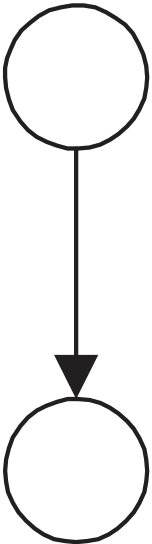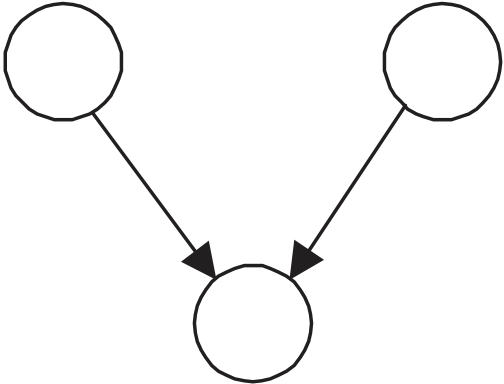Rating : ⭐⭐⭐⭐⭐
Price : \$10.99
Language:EN
Pages: 2

# Figure con-structed the dag using causal edges1.3. LARGE INSTANCES / BAYESIAN NETWORKS 41

Test

P(v1) = 5/13 P(s1) = 8/13

V S

42 CHAPTER 1. INTRODUCTION TO BAYESIAN NETWORKS

P(h1) = .2

P(l1|h1) = .003
P(l1|h2) = .00005

F

C

Example 1.32 Recall the situation discussed at the beginning of this section where we were concerned with the joint probability distribution of smoking his-tory (H), bronchitis (B), lung cancer (L), fatigue (F), and chest X-ray (C). Figure 1.1, which appears again as Figure 1.10, shows a Bayesian network con-taining those variables in which the conditional distributions were estimated from actual data.

Does the Bayesian network in the previous example contain the actual rel-ative frequency distribution of the variables? Example 1.31 illustrates that if we develop a Bayesian network from an arbitrary DAG and the conditionals of a probability distribution P relative to that DAG, in general the resultant Bayesian network does not contain P. Notice that, in Figure 1.10 we con-structed the DAG using causal edges. For example, there is an edge from H to L because smoking causes lung cancer. In the next section, we argue that if we construct a DAG using causal edges we often have a DAG that satisfies the Markov condition with the relative frequency distribution of the variables. Given this, owing to Theorem 1.4, the relative frequency distribution of the variables in Figure 1.10 should satisfy the Markov condition with the DAG in

How It Works